# ISEE Lower Level Quantitative : How to find the area of a parallelogram

## Example Questions

### Example Question #1 : Geometric Measurement: Understanding Concepts Of Area And Relating Area To Multiplication And To Addition

How many square units make up the area of the shape below?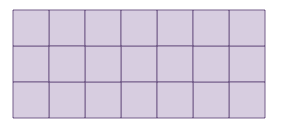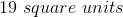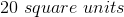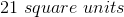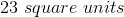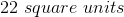Explanation:

The shape is made up of unit squares. We can count the number of squares within the shape to find the area.

There aresquares within the shape.

### Example Question #61 : Plane Geometry

How many square units make up the area of the shape below?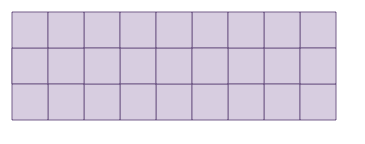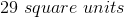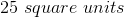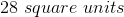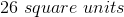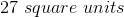Explanation:

The shape is made up of unit squares. We can count the number of squares within the shape to find the area.

There aresquares within the shape.

### Example Question #1 : Geometric Measurement: Understanding Concepts Of Area And Relating Area To Multiplication And To Addition

How many square units make up the area of the shape below?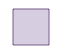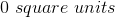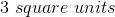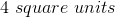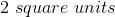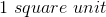Explanation:

The shape is made up of unit squares. We can count the number of squares within the shape to find the area.

There aresquare within the shape.

### Example Question #1 : Geometric Measurement: Understanding Concepts Of Area And Relating Area To Multiplication And To Addition

How many square units make up the area of the shape below?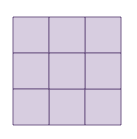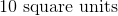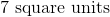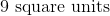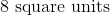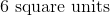Explanation:

The shape is made up of unit squares. We can count the number of squares within the shape to find the area.

There aresquares within the shape.

### Example Question #411 : Quadrilaterals

How many square units make up the area of the shape below?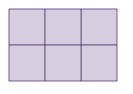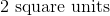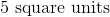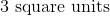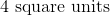Explanation:

The shape is made up of unit squares. We can count the number of squares within the shape to find the area.

There aresquares within the shape.

### Example Question #1541 : Common Core Math: Grade 3

How many square units make up the area of the shape below?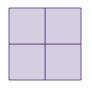Explanation:

The shape is made up of unit squares. We can count the number of squares within the shape to find the area.

There aresquares within the shape.

### Example Question #11 : Unit Squares Used To Measure Area: Ccss.Math.Content.3.Md.C.5a

How many square units make up the area of the shape below?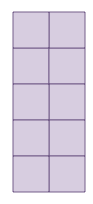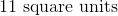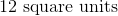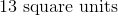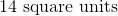Explanation:

The shape is made up of unit squares. We can count the number of squares within the shape to find the area.

There aresquares within the shape.

### Example Question #61 : Plane Geometry

How many square units make up the area of the shape below?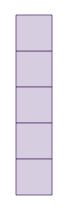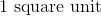Explanation:

The shape is made up of unit squares. We can count the number of squares within the shape to find the area.

There aresquares within the shape.

### Example Question #1 : Apply Area And Perimeter Formulas For Rectangles: Ccss.Math.Content.4.Md.A.3

What is the length of a rectangular room with a perimeter of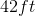and a width of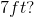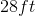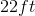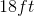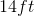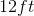Explanation: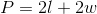We have the perimeter and the width, so we can plug those values into our equation and solve for our unknown.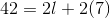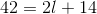Subtractfrom both sides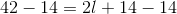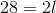Divideby both sides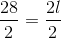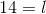### Example Question #2 : Apply Area And Perimeter Formulas For Rectangles: Ccss.Math.Content.4.Md.A.3

What is the length of a rectangular room with a perimeter of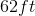and a width of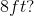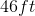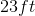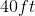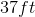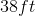Explanation:We have the perimeter and the width, so we can plug those values into our equation and solve for our unknown.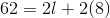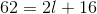Subtractfrom both sides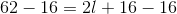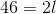Divideby both sides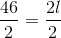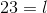### All ISEE Lower Level Quantitative Resources